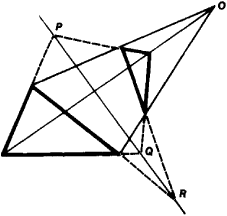# Desargues's Theorem

Also found in: Wikipedia.
The following article is from The Great Soviet Encyclopedia (1979). It might be outdated or ideologically biased.

## Desargues’s Theorem

a theorem in projective geometry established by the French mathematician G. Desargues. Desargues’s theorem states (see Figure 1) that if the corresponding sides of two triangles intersect the points P, Q, and R lying on the same straight line, then the straight lines connecting the corresponding vertices intersect at a single point O. The inverse theorem is also true: if the straight lines connecting the corresponding vertices of two triangles pass through a single point, then the points of intersection P, Q, and R of the corresponding sides of these triangles lie on the same straight line. The essence of Desargues’s theorem concerns the relative positions of straight lines and is not related to measurements. However, as was established by D. Hilbert, this theorem cannot be proved in plane geometry without involving metric axioms (so-called “non-Desargues” plane geometries exist, in which all projective axioms are fulfilled but Desargues’s theorem is not valid). For this reason, in an axiomatic construction of plane projective geometry, the condition of Desargues’s theorem is accepted as an axiom.Figure 1

E. G. POZNIAK